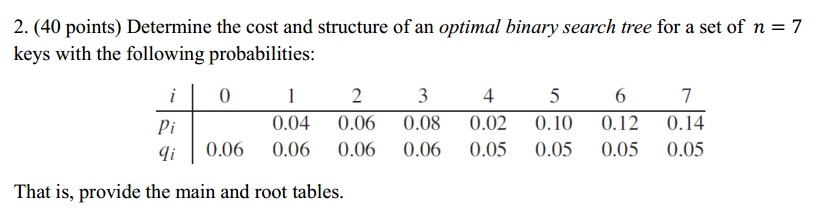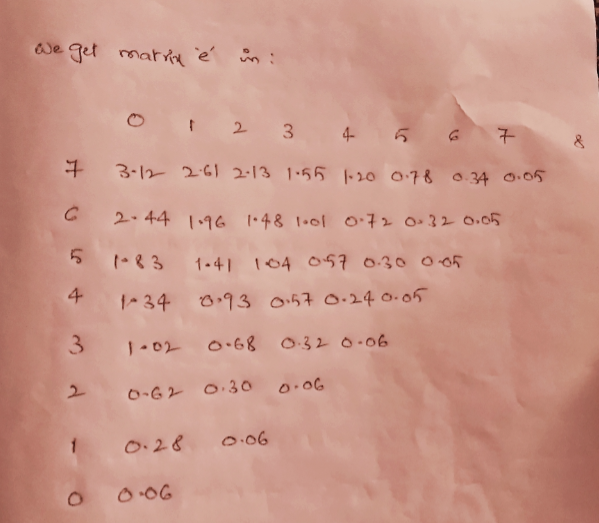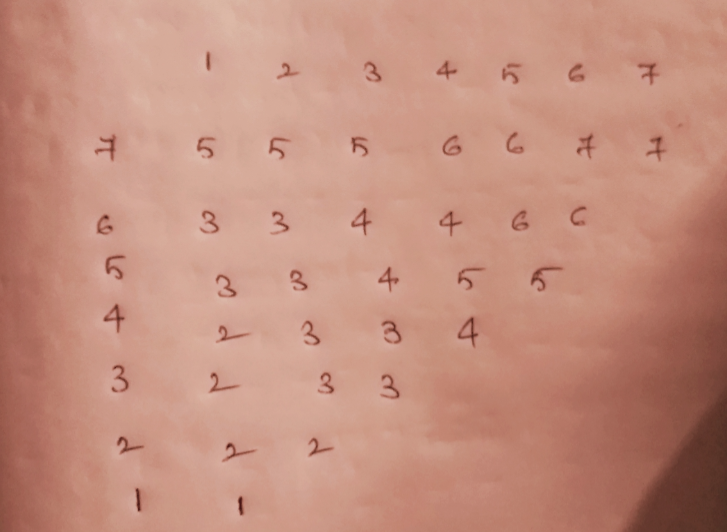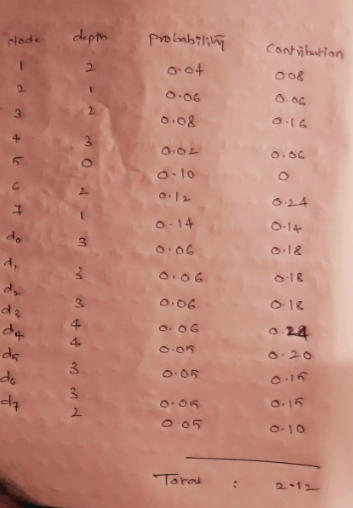# Answered! Determine the cost and structure of an optimal binary search tree for a set of n = 7 keys with the following…Determine the cost and structure of an optimal binary search tree for a set of n = 7 keys with the following probabilities: That is, provide the main and root tables.

We have to solve this by using OPTIMAL-BST(p, q, 7). As per this algorithm it returns two

matrices, e and root. Matrix e is abput expected search costs and root allows us to construct optimal binary search tree.

Don't use plagiarized sources. Get Your Custom Essay on
Answered! Determine the cost and structure of an optimal binary search tree for a set of n = 7 keys with the following…
GET AN ESSAY WRITTEN FOR YOU FROM AS LOW AS \$13/PAGE

The e matrix e is as follows:Root matrix is :Then the optimal binary search tree is as follows:

 5 is the root d3 is the left child of 4 2 is the left child of 5 d4 is the right child of 4 of 1 is the left child of 2 7 is the right child of 5 d0 is the left child of 1 6 is the left child of 7 d1 is the right child of 1 d5 is the left child of 6 3 is the right child of 2 d6 is the right child of 6 d2 is the left child of 3 d7 is the right child of 7 4 is the right child of 3

Finally calculate the expected search cost.